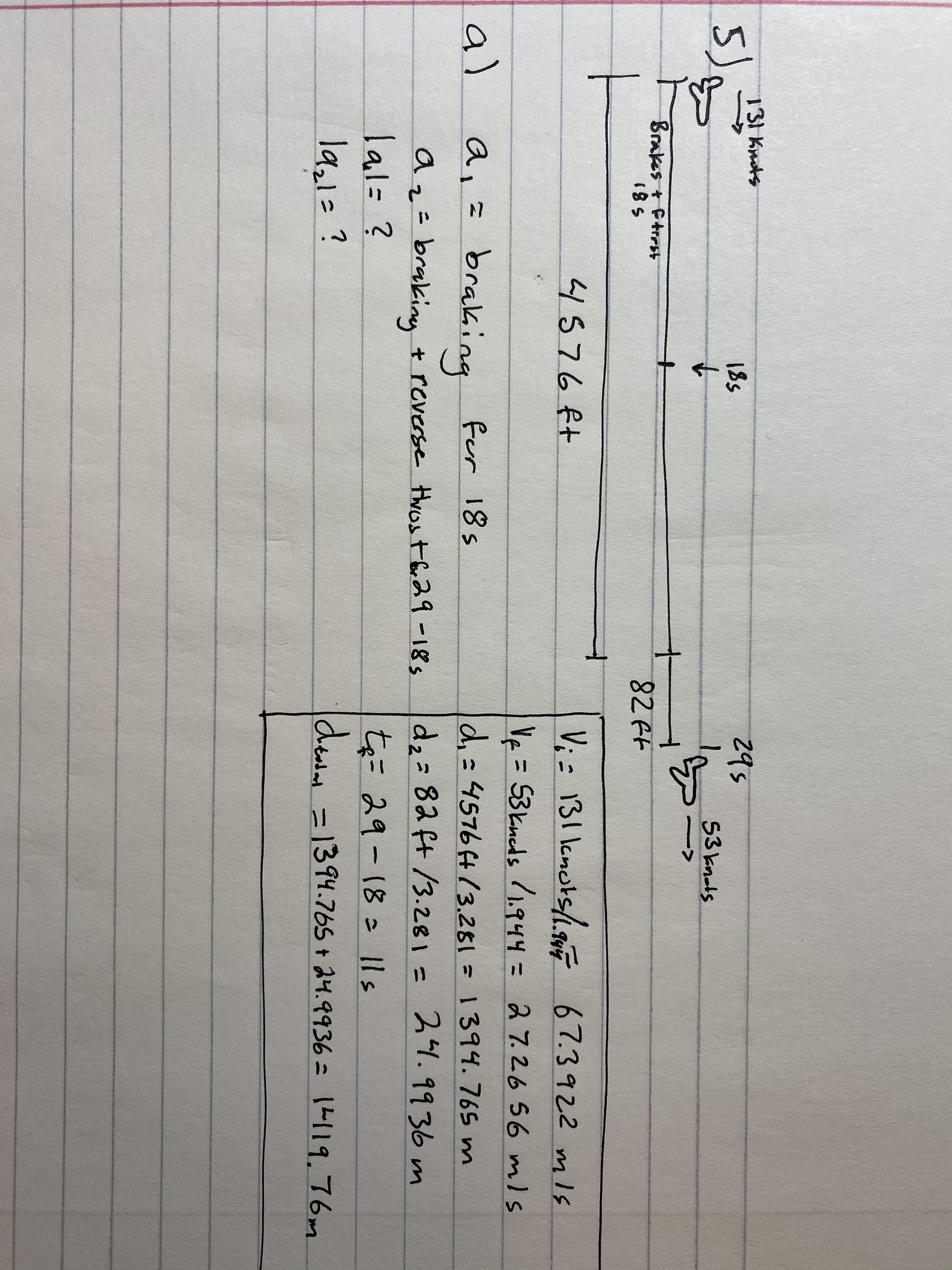# 131 Knots2951855)53 knolsBrakes t Ftrest18582 Ft4576ftV; = 131 lcnots/.gF67.3922 mIsVe= 53kneds /1.944= 27.2656 mlsalbrakingd,=45764(3.281=1394.765 m24.9936 mfor 18sdz= 82 ft /3.281=az= brakiny + reverse thost br29 -18slal=?la,1=?te= 29-18 1lsde =1394.765 + 24.9936 = 1L119.76m

Question
27 views

A plane touches down with 4576 ft of runway and an 82 foot safety zone.  The planes lands going 131 knots.  It brakes for 18 sec before initiating reverse thrust for an additional 11 sec.  After the total 29 sec the plane leaves the safety zone and hits a car. (Diagram)

What is the acceleration (a1) for the first 18 seconds?

What is the second acceleration (a2) for the 11 seconds?help_outlineImage Transcriptionclose131 Knots 295 185 5) 53 knols Brakes t Ftrest 185 82 Ft 4576ft V; = 131 lcnots/.gF 67.3922 mIs Ve= 53kneds /1.944= 27.2656 mls al braking d,=45764(3.281=1394.765 m 24.9936 m for 18s dz= 82 ft /3.281= az= brakiny + reverse thost br29 -18s lal=? la,1=? te= 29-18 1ls de =1394.765 + 24.9936 = 1L119.76m fullscreen
check_circle

Step 1

the expression to calculate the acceleration is,

Step 2

substitute the known values,

Step 3

b)

the relation between the inital ...

### Want to see the full answer?

See Solution

#### Want to see this answer and more?

Solutions are written by subject experts who are available 24/7. Questions are typically answered within 1 hour.*

See Solution
*Response times may vary by subject and question.
Tagged in

### Physics